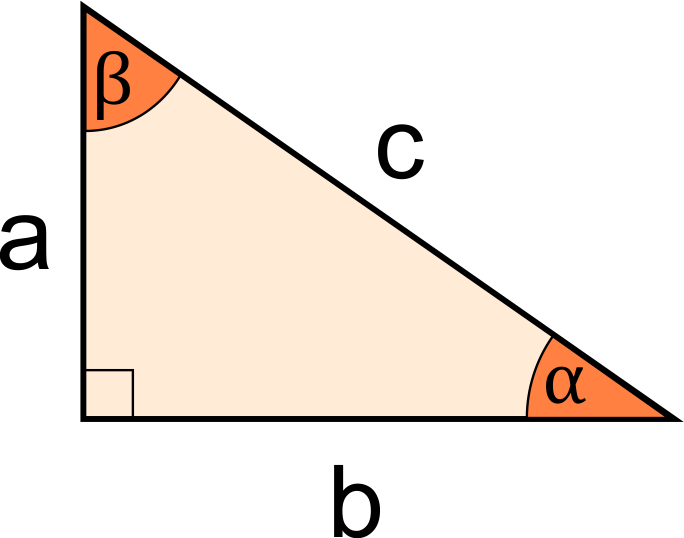# Angle of Right Triangle Calculator

Created by Kenneth Alambra
Last updated: May 25, 2022

This angle of a right triangle calculator will help you determine what the angles of a right triangle are - that is, if you know the measurements of at least two of its sides or at least one of its sides together with its area. Keep on reading to learn about:

• How to find the angles of a right triangle;
• How to use this calculator; and
• Our other triangle calculators.

## How to find the angles of a right triangle?

Since a triangle has a total of 180° for its internal angles, having one corner have an angle measurement of 90° means that the remaining two other angles should have a total of 90°. With that said, we can say that the two remaining angles $\alpha$ and $\beta$ are complementary angles. In equation form, we express that as:

$\alpha + \beta = 90\degree$

So, if we know one of these two angles, how to find the missing angles of a right triangle is as easy as subtracting our known angle from 90° 🙂.

On the other hand, if we do not know any of the two angles, we can still find them with the help of some basic trigonometric functions.

To find angle $\alpha$, we can use the following equations:

• $\alpha = arctan(a / b)$
• $\alpha = arccos(b / c)$
• $\alpha = arcsin(a / c)$

To determine angle $\beta$, here are the equations we can use:

• $\beta = arctan(b / a)$
• $\beta = arccos(a / c)$
• $\beta = arcsin(b / c)$

With those equations, we can see that if we have the measurements of at least two sides of our right triangle, we can determine what the angles of a right triangle are.

What if you only know the measurement of one leg? For that, you should at least also know the area of your right triangle to derive the other side of your triangle using one of these equations:

To find $a$ given the $\text{area}$ and $b$:

$a = 2 \times \text{area} / b$

To find $b$ given the \text{area} and $a$:

$b = 2 \times \text{area} / a$

Then you can use the formulas we previously discussed to find the missing angles of your right triangle.

The fun part is that we have already utilized these equations in this calculator for your convenience. Head to the next section of this text to learn how to use this tool for your angle of right triangle calculations 🙂.

🙋 Note that we cannot solve for the angles of a right triangle if we only know its hypotenuse $c$ and its $\text{area}$ because different right triangles can have the same hypotenuse but with different areas.

## How to use our angle of right triangle calculator

To use our angle of a right triangle calculator, all you have to do is enter any two known sides of your right triangle. That could be the measurements of your right triangle's two legs ($a$ and $b$), or one leg measurement and the hypotenuse ($a$ and $c$, or $b$ and $c$). You can also enter one of your triangle's sides ($a$, $b$, or $c$) and the $\text{area}$ of your right triangle.

Upon doing so, you'll instantly see your right triangle's angles: both angle $\alpha$ and angle $\beta$. It's that simple!

If you want to expand your knowledge about triangles even further, here is a list of our other triangle calculators that you can check out:

## FAQ

### What are the angles of a right triangle?

Let's say a right triangle has an area of 20 cm² and one of its legs equal to 4 cm. To find the angles of this right triangle:

1. First, we find the other side of the triangle using this equation: b = 2 × area / a, where a = 4 cm. We'll then have b = 2 × 20 cm² / 4 cm = 10 cm.
2. Then we calculate the angle α opposite a to be equal to α = arctan(a / b): α = arctan(4 cm / 10 cm) = 21.8°.
3. Finally, we can subtract α from 90° to find β: β = 90° - 21.8° = 68.2°.

### What are the 3 angles of a right triangle?

The three angles of a right triangle are one 90° angle and two acute angles that are complementary angles. That means if a right triangle has one of its angles equal to 30°, its other angle would be 60°. On the other hand, if a right triangle is an isosceles triangle, its acute angles should both be 45° to meet this condition.

Kenneth Alambraa
in
b
in
c
in
Area
in²
Results
Angle α
deg
Angle β
deg
People also viewed…

### Absolute value

Calculate the absolute value of any number with this simple calculator. On top of that, we have provided tricks and tips to help solve absolute value equations and inequalities as well as with how to plot absolute value functions.